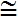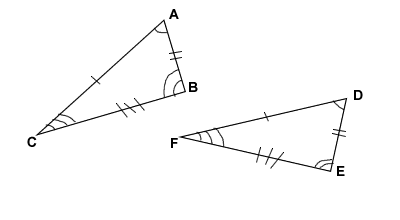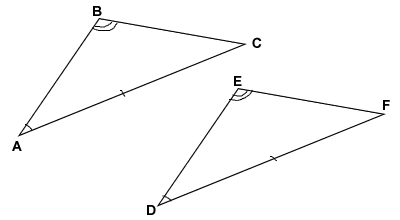# Congruent Means "Identical In Form"

#### The Definition

Before we even start, let me remind you that congruent means "the same" in geometry. As written above, it means "identical in form." If you cut two identical triangles from a sheet of paper, and couldn't tell them apart based on size or shape, they would be congruent.

We use the following symbol to indicate congruence:It means not only are the two figures the same shape (~), but they have the same size (=).

#### Going into more detail...

Two figures that are congruent have what are called corresponding sides and corresponding angles. That means that parts that are the same and would match up if you stacked the two figures. It is important to recognize that in a congruent triangle, each part of it is also obviously congruent. If two triangles are congruent, then naturally all the sides are angles are also congruent with their corresponding pair.

In every congruent triangle:

• (1) there are 3 sets of congruent sides and
• (2) there are 3 sets of congruent angles.

Below we have two triangles: triangle ABC and triangle DEF.NOTE: The corresponding congruent sides are marked with small straight line segments called hash marks. The corresponding congruent angles are marked with arcs. See picture above.

For those same two triangles, ABC and DEF, we know the following:

• (1) line segment AB isto line segment DE.
• (2) line segment BC isto line segment EF.
• (3) line segment AC isto line segment DF.
• (4) angle A isto angle D.
• (5) angle B isto angle E.
• (6) angle C isto angle F.

Notice that each one of these properties makes common sense. If you have two identical triangles, it should be obvious that their angles are identical. Nonetheless, these are still important facts. Why? Because now all we have to do is prove that two triangles are congruent. We don't have to worry about proving the sides or angles are congruent. This can be very useful.

#### Proving Congruency

If we need to prove that two triangles are congruent, we have five different methods:

1. SSS (side side side) = If three sides of one triangle are congruent to three sides of another triangle, the triangles are congruent.

2. SAS (side angle side) = If two sides and the angle in between are congruent to the corresponding parts of another triangle, the triangles are congruent.

3. ASA (angle side angle) = If two angles and the side in between are congruent to the corresponding parts of another triangle, the triangles are congruent.

4. AAS (angle angle side) = If two angles and the non-included side of one triangle are congruent to the corresponding parts of another triangle, the triangles are congruent.

5. HL (hypotenuse leg) = If the hypotenuse and leg of one right triangle are congruent to the corresponding parts of another right triangle, the right triangles are congruent. Remember that if we know two sides of a right triangle we know the third side anyway, so this is really just SSS.

NOTE 1: AAA works fine to show that triangles are the same SHAPE (similar), but does NOT work to show congruence. You can draw 2 equilateral triangles that are the same shape but not the same size.

NOTE 2: The Angle Side Side theorem (yes, we all know what it spells) does NOT necessarily work.

I will now show you the basics of proving (showing) that two triangles are congruent. However, I will go over this again in more detail in future geometric proof lessons. For example, later on, I will show you how to use the statements versus reasons charts but for now, I will stick to the basics.

#### Example A:

Is triangle ABC congruent to triangle DEF?In the pictures we have:

angle Aangle D.
angle Bangle E.
side ACside DF.

Conclusion: triangle ABCtriangle DEF by the AAS theorem.

#### Example B:

Is triangle ABC congruent to triangle XYZ?What do we know from this picture? We see an angle and two sides that are congruent. However, there is no congruence for Angle Side Side. Therefore we can't prove that the triangles are congruent.

It's important to note that the triangles COULD be congruent, and in fact in the diagram they are the same. But I could have manipulated the triangles to make them non-congruent with the same Angle Side Side relationship. All you know is that you need more information to decide if they are congruent or not.

A lesson provided by Mr. Feliz